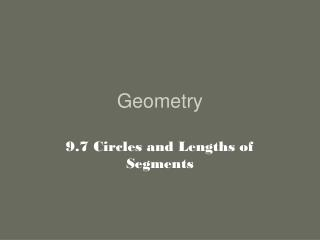DownloadDownload PresentationGeometry

# Geometry

Download Presentation## Geometry

- - - - - - - - - - - - - - - - - - - - - - - - - - - E N D - - - - - - - - - - - - - - - - - - - - - - - - - - -
##### Presentation Transcript

1. Geometry 9.7 Circles and Lengths of Segments

2. Q S X R P Theorem • When two chords intersect inside a circle, the product of the segments of one chord equals the product of the segments of the other chord. 4 3 x 8 6 8 x 3 = 6 x 4

3. D A B P C Example Another way to solve would be to think of two numbers that multiply to 36 and add to 15. b. If AP = 4, PB = 9, and CD = 15, find CP. x 4 P 9 15 - x 9 x 4 = x(15 – x) 36 = 15x – x2 x2 – 15x + 36 = 0 - - (x )(x ) = 0 3 12 CP is either 12 or 3. x – 3 = 0 or x – 12 = 0 If it were given that the figure was drawn to scale, then CP = 12. x = 3 or x = 12

4. E D F G H Theorem • When two secant segments are drawn to a circle from an external point, the product of one secant segment and its external segmentequalsthe product of the other secant segment and its external segment. FD x FE = FH x FG External x Whole Thing = External x Whole Thing

5. P P S S T T Q Q R R Example a. If PQ = 9, QR = 3, and TR = 4, then SR = ____. b. If PQ = 11, SR = 12, and TR = 5, find PR. 9 3(12) = 4x 12 36 = 4x x PR = x + 11 = (4) + 11 = 15 4 9 = x 3 RS = 9 x(x + 11) = 5(12) x2 + 11x = 60 x2 + 11x – 60 = 0 11 x + 11 - + (x )(x ) = 0 4 15 12 5 x x – 4 = 0 or x + 15 = 0 x = 4 or x = -15 x must be positive

6. A P B C Theorem • When a secant segment and a tangent segment are drawn to a circle from an external point, the product of the secant segment and its external segment is equal to the square of the tangent segment. PB x PC = (PA)2 External x Whole Thing = (Tangent)2

7. T T S S R R Q Q Example 25 5 a. If RT = 25 and RS = 5, find QR. b. IF QR = 6 and ST = 9, find RS. x x2 = 5(25) x2 = 125 x= 5√5 x + 9 QR= 5√5 9 x x(x + 9) = 62 x2 + 9x = 36 x must be positive. x2 + 9x – 36 = 0 6 (x )(x ) = 0 - + 3 12 x – 3 = 0 or x + 12 = 0 RS = 3 x = 3 or x = -12

8. R U T Q S • Complete. • If RQ=8, QS = 6, and TQ = 16, then QU = ____. • 2. If RS =13, RQ = 7, and QU = 3, then TQ = ____. • 3. If TU = 10√2, QU = 2√2 , and RQ = 8, then RS ____. • 4. If RS = 17, TQ = 18, and QU = 4, then RQ = ___. 3 14 Q 12 8 or 9 Who can fill in the picture on the front board?

9. D E F G H Complete. 5. If DF = 17, EF = 3, and GF = 6, then HF = ____. 6. If HG = 8, GF = 7, and DF = 21, then EF = ____. 7. If GF = 8, HG = 10, and DE = 18, then EF = ____. 8. If DF = 25, EF = 4, and HG = GF = x, then x = ____. 8½ 5 6 5√2

10. 15 x 4 5 8 12 16 x x 3x x Secants and tangents are shown. Find the value of x. 9) 10) 11) 12) x = 6 x = 3 x = 9 x = 4

11. HW • Be nice to someone, take the trash out • P. 363-366 WE(1-9, 13-20, 24)